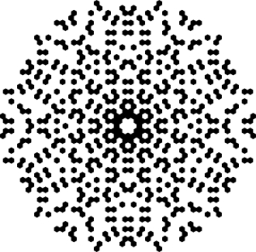# Decompose 37101

Decompose the number of primes and find its digit sum:
180,
232,
460,
240,
365,

a =  180
b =  232
c =  460
d =  240
e =  365
c1 =  9
c2 =  7
c3 =  10
c4 =  6
c5 =  14

### Step-by-step explanation:Did you find an error or inaccuracy? Feel free to write us. Thank you!

Tips for related online calculators
Do you want to perform natural numbers division - find the quotient and remainder?